## How to Calculate and Solve for Freezing Time | Solidification of MetalsThe image above represents freezing time.

To compute for freezing time, three essential parameters are needed and these parameters are Thickness of Solidified Metal (M), Constant (β) and Heat Diffusivity (α).

The formula for calculating freezing time:

t = / 4β²α

Where:

t = Freezing Time
M = Thickness of Solidified Metal
β = Constant
α = Heat Diffusivity

Let’s solve an example;
Find the freezing time when the thickness of solidified metal is 8, the constant is 10 and the heat diffusivity is 2.

This implies that;

M = Thickness of Solidified Metal = 8
β = Constant = 10
α = Heat Diffusivity = 2

t = / 4β²α
t = / 4(10)²(2)
t = 64 / 4(100)(2)
t = 64 / 800
t = 0.08

Therefore, the freezing time is 0.08 s.

Calculating the Thickness of Solidified Metal when the Freezing Time, the Constant and the Heat Diffusivity is Given.

M = t x 4β²α

Where:

M = Thickness of Solidified Metal
t = Freezing Time
β = Constant
α = Heat Diffusivity

Let’s solve an example;
Find the thickness of solidified metal when the freezing time is 21, the constant is 9 and the heat diffusivity is 2.

This implies that;

t = Freezing Time = 21
β = Constant = 9
α = Heat Diffusivity = 2

M = t x 4β²α
M = 21 x 4(9²)(2)
M = 21 x 4(81)(2)
M = 21 x 4(162)
M = 21 x 648
M = 13668

Therefore, the thickness of solidified metal is 13668.

## How to Calculate and Solve for Thickness of Solidifying Metals | Solidification of Metals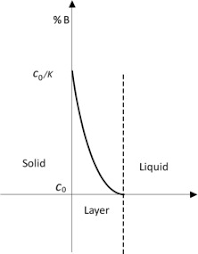The image above represents thickness of solidifying metals.

To compute for thickness of solidifying metals, six essential parameters are needed and these parameters are Melting Temperature of Metal (Tm), Initial Mould Temperature (To), Heat Diffusivity (α), Time (t), Density (ρ’) and Latent Heat of Fusion (Hf).

The formula for calculating thickness of solidifying metals:

M = 2(Tm – To)√(α)√(t) / √(π)ρ’Hf

Where:

M = Thickness of Solidifying Metal
Tm = Melting Temperature of Metal
To = Initial Mould Temperature
α = Heat Diffusivity
t = Time
ρ = Density
Hf = Latent Heat of Fusion

Let’s solve an example;
Find the thickness of solidifying metal when the melting temperature of metal is 4, the initial mould temperature is 8, the heat diffusivity is 2, the time is 6, the density is 3 and the latent heat of fusion is 7.

This implies that;

Tm = Melting Temperature of Metal = 4
To = Initial Mould Temperature = 8
α = Heat Diffusivity = 2
t = Time = 6
ρ = Density = 3
Hf = Latent Heat of Fusion = 7

M = 2(Tm – To)√(α)√(t) / √(π)ρ’Hf
M = 2(4 – 8)√(2)√(6) / √(π)(3)(7)
M = 2(-4)(1.414)(2.449) / (1.772)(3)(7)
M = -27.712 / 37.22
M = -0.744

Therefore, the thickness of solidifying metal is -0.744 m.

## How to Calculate and Solve for Heat Diffusivity | Solidification of Metals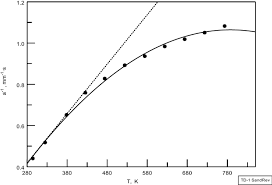The image above represents heat diffusivity.

To compute for heat diffusivity, three essential parameters are needed and these parameters are Thermal Conductivity (K), Density (ρ) and Heat Capacity at Constant Pressure (cp).

α = Kρcp

Where:

α = Heat Diffusivity
K = Thermal Conductivity
ρ = Density
cp = Heat Capacity at Constant Pressure

Let’s solve an example;
Find the heat diffusivity when the thermal conductivity is 10, the density is 12 and the heat capacity at constant pressure is 18.

This implies that;

K = Thermal Conductivity = 10
ρ = Density = 12
cp = Heat Capacity at Constant Pressure = 18

α = Kρcp
α = (10)(12)(18)
α = 2160

Therefore, the heat diffusivity is 2160 m²/s.

Calculating the Thermal Conductivity when the Heat Diffusivity, the Density and the Heat Capacity at Constant Pressure is Given.

K = α / ρcp

Where:

K = Thermal Conductivity
α = Heat Diffusivity
ρ = Density
cp = Heat Capacity at Constant Pressure

Let’s solve an example;
Find the thermal conductivity when the heat diffusivity is 15, the density is 10 and the heat capacity at constant pressure is 14.

This implies that;

α = Heat diffusivity = 15
ρ = Density = 10
cp = Heat Capacity at Constant Pressure = 14

K = α / ρcp
K = 15 / 10 (14)
K = 15 / 140
K = 0.107

Therefore, the thermal conductivity is 0.107.

Calculating the Density when the Heat Diffusivity, the Thermal Conductivity and the Heat Capacity at Constant Pressure is Given.

ρ = α / K x cp

Where:

ρ = Density
α = Heat Diffusivity
K = Thermal Conductivity
cp = Heat Capacity at Constant Pressure

Let’s solve an example;
Find the density when the heat diffusivity is 18, the thermal conductivity is 12 and the heat capacity at constant pressure is 4.

This implies that;

α = Heat Diffusivity = 18
K = Thermal Conductivity = 12
cp = Heat Capacity at Constant Pressure = 4

ρ = α / K x cp
ρ = 18 / 12 x 4
ρ = 18 / 48
ρ = 0.375

Therefore, the density is 0.375.

Calculating the Heat Capacity at Constant Pressure when the Heat Diffusivity, the Thermal Conductivity and the Density is Given.

cp = α / K x ρ

Where:

cp = Heat Capacity at Constant Pressure
α = Heat Diffusivity
K = Thermal Conductivity
ρ = Density

Let’s solve an example;
Find the heat capacity at constant pressure when the heat diffusivity is 10, the thermal conductivity is 20 and the density is 6.

This implies that;

α = Heat Diffusivity = 10
K = Thermal Conductivity = 20
ρ = Density = 6

cp = α / K x ρ
cp = 10 / 20 x 6
cp = 10 / 120
cp = 0.083

Therefore, the heat capacity at constant pressure is 0.083.

Nickzom Calculator – The Calculator Encyclopedia is capable of calculating the heat diffusivity.

To get the answer and workings of the heat diffusivity using the Nickzom Calculator – The Calculator Encyclopedia. First, you need to obtain the app.

You can get this app via any of these means:

To get access to the professional version via web, you need to register and subscribe for NGN 2,000 per annum to have utter access to all functionalities.
You can also try the demo version via https://www.nickzom.org/calculator

Apple (Paid) – https://itunes.apple.com/us/app/nickzom-calculator/id1331162702?mt=8
Once, you have obtained the calculator encyclopedia app, proceed to the Calculator Map, then click on Materials and Metallurgical under Engineering.Now, Click on Solidification of Metal under Materials and Metallurgical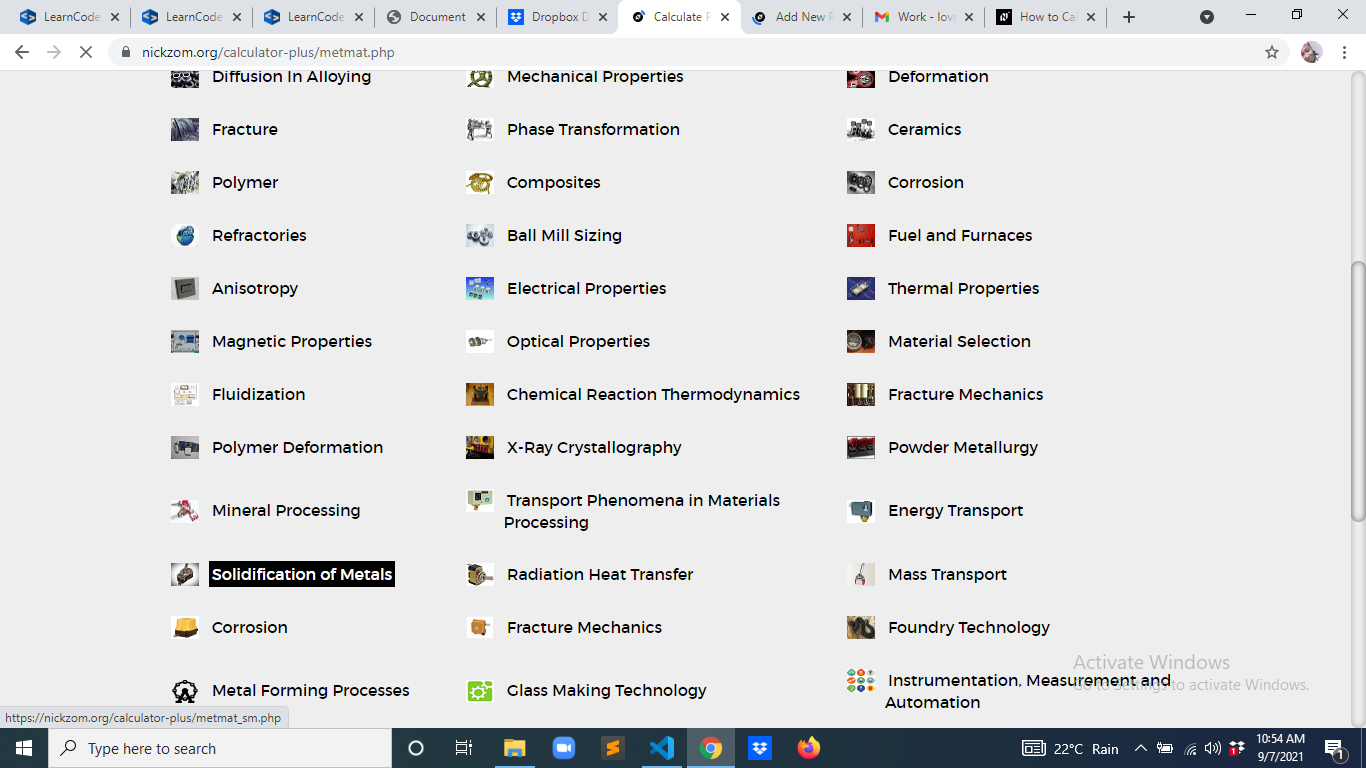Now, Click on Heat Diffusivity under Solidification of Metal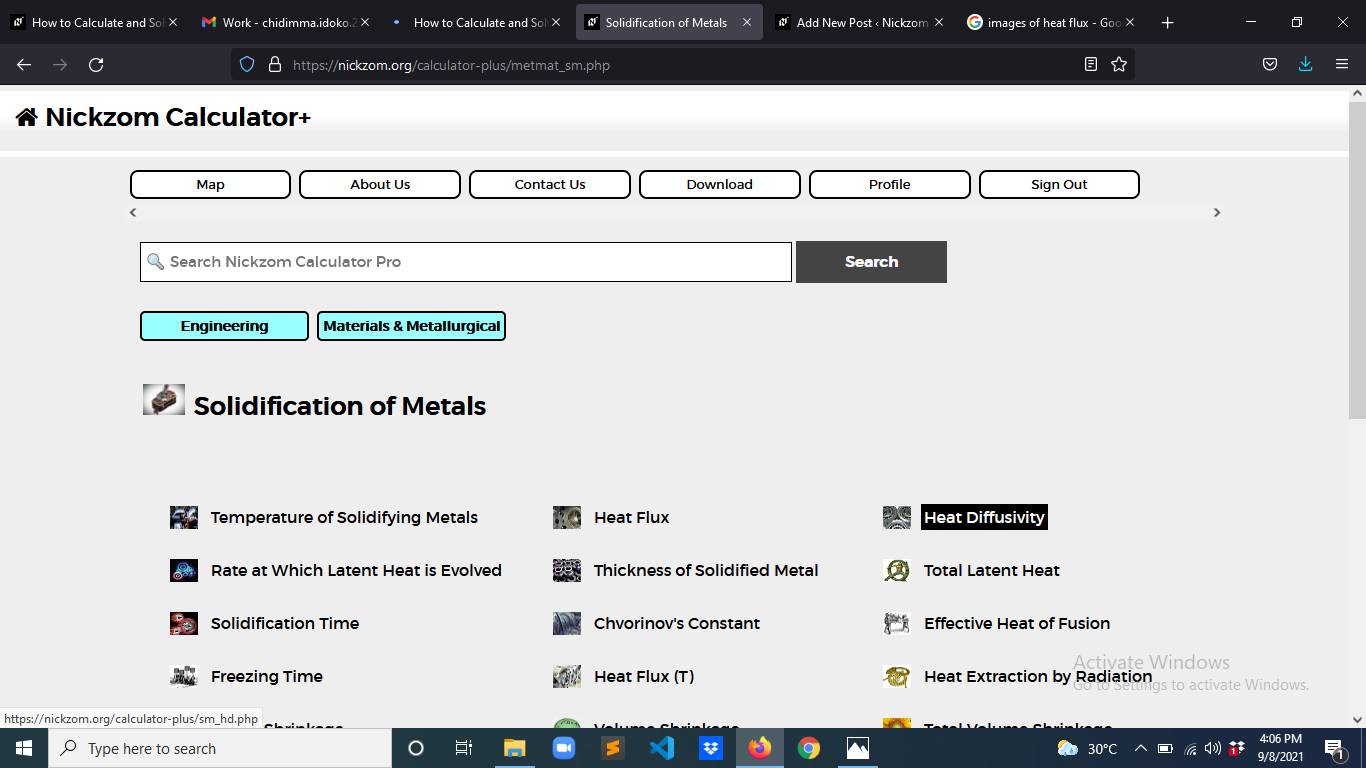The screenshot below displays the page or activity to enter your values, to get the answer for the heat diffusivity according to the respective parameter which is the Thermal Conductivity (K), Density (ρ) and Heat Capacity at Constant Pressure (cp).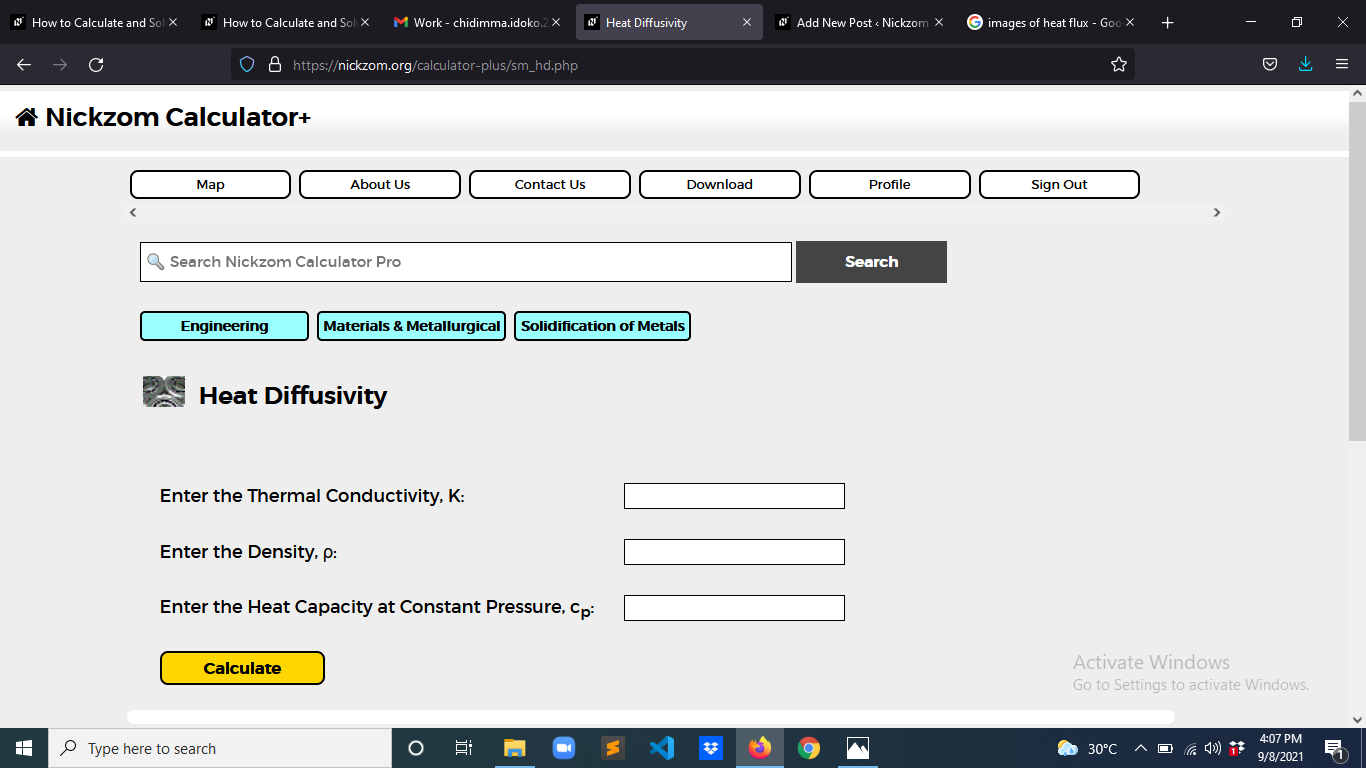Now, enter the values appropriately and accordingly for the parameters as required by the Thermal Conductivity (K) is 10, Density (ρ) is 12 and Heat Capacity at Constant Pressure (cp) is 18.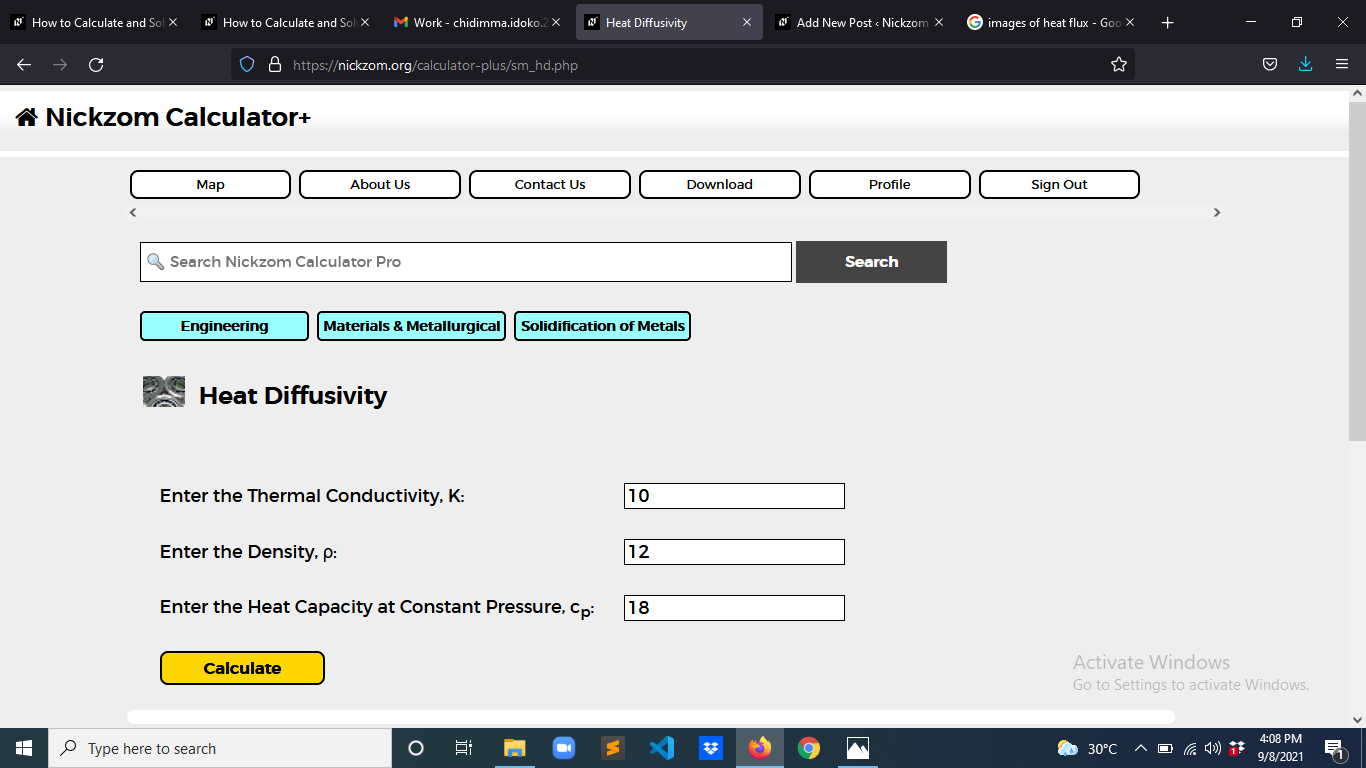Finally, Click on Calculate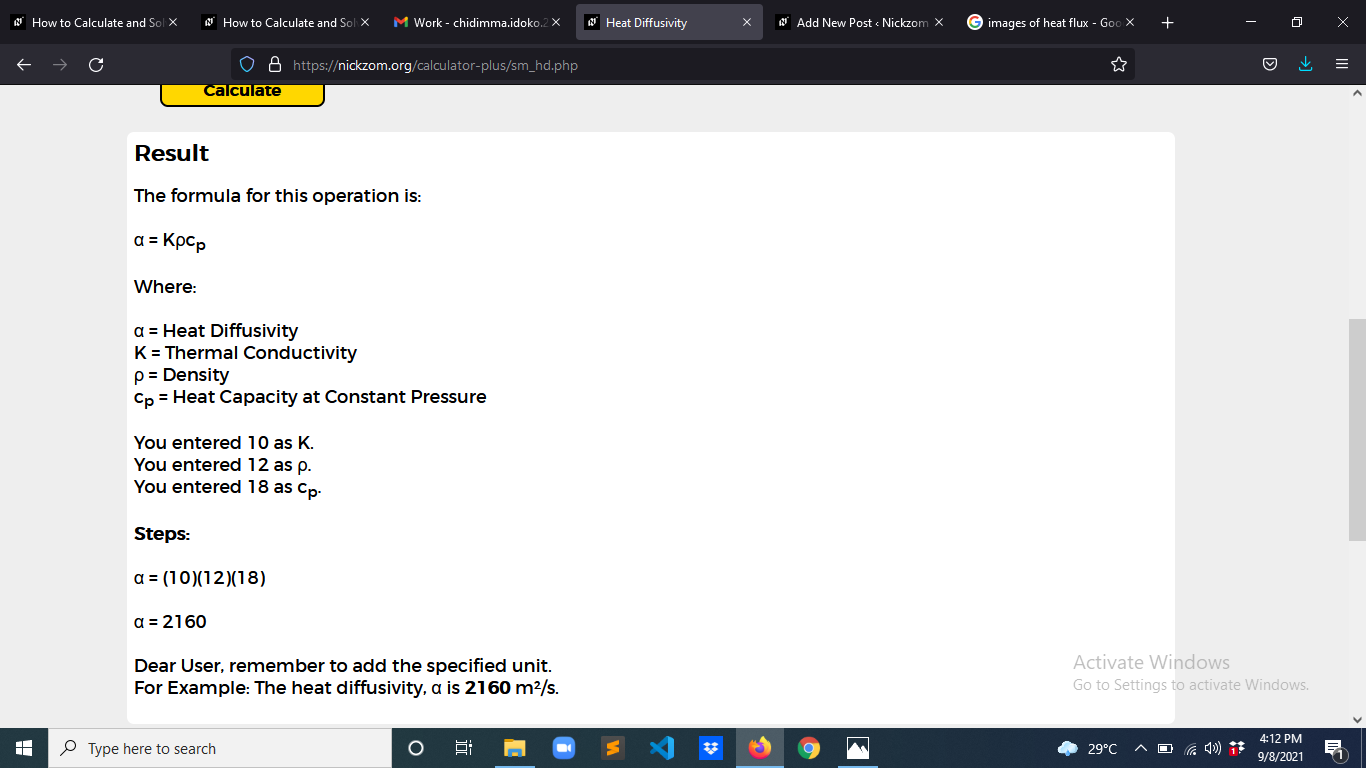As you can see from the screenshot above, Nickzom Calculator– The Calculator Encyclopedia solves for the heat diffusivity and presents the formula, workings and steps too.